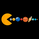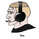6531 views
Logistic Map Equation - The logistic map connects fluid convection, neuron firing, the Mandelbrot set and so much more.

This study is an attempt to apply Logistic Map Equation in Trading

Logistic Map Equation

Xn+1 = r * Xn * (1 - Xn)

Where,
r - growth rate
Xn - percentage of theoretical maximum of measured event (from 0 to 1)
(1 - Xn) - represents constraints of the environment, presents the idea of negative feedback

For trading the measured event will be the price of the instrument (price is commonly reffered as source in mathematicall forumlations),
hence
r - growth rate can be expressed as => change(source, length) / source, expressing r in such manner mades the equation dynamic with regards to the growth rate
Xn - percentage of theoretical maximum of the price for given duration can be expressed as => source / highest(length)

Putting pieces together we are ready to plot

Printed alone does not seem to provide much useful visualization for trading, in fact not easy to interpret especially when the market is an uptrend

What it has numerically,
Provides a ratio, where sudden changes are much more reflected thanks to negative feedback nature of the logistic equation.

As we know moving average indicators are lagging and the logistic map may fit here to reduce the lag

With this study you will find application of Logistic Map Equation with combination of Exponential Moving Average ( EMA )

Logistic EMA (LEMA) and LEMA COLORS
one line with user defined periods of length, where the colors of the line will change automatically depending where the value is compared to 50-100-200 moving average

Multiple LEMAs : optional – three fixed lenght of 50-100-200 period lines

LEMA Signals
Various signals are added by using LEMA and applying some common market approaches. Use with caution and with conjunction of other indicators

Thanks to @allanster for the idea
A fascinating YouTube video explaining the logistic map - “This equation will change how you see the world (the logistic map)”

Disclaimer:

The script is for informational and educational purposes only. Use of the script does not constitute professional and/or financial advice. You alone have the sole responsibility of evaluating the script output and risks associated with the use of the script. In exchange for using the script, you agree not to hold dgtrd TradingView user liable for any possible claim for damages arising from any decision you make based on use of the script

Release Notes: minor fix
Release Notes: enchamnent :

- lema plotting now is made of two lines, the first one as explined above, the second line colors calculated based on high low values

- lema values optimized

- macd removed form probable trade singnals and instead trend dicrection calculated based on high low moving averages added
Open-source script

In true TradingView spirit, the author of this script has published it open-source, so traders can understand and verify it. Cheers to the author! You may use it for free, but reuse of this code in a publication is governed by House Rules. You can favorite it to use it on a chart.

Want to use this script on a chart?blackcat1402
thank you @blackcat1402, logistic map equation is fascinating ;-)